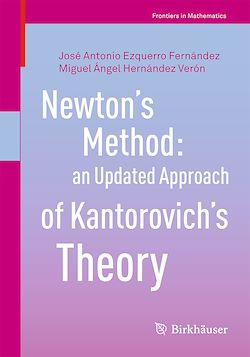166 Pages
English

# Newton’s Method: an Updated Approach of Kantorovich’s Theory

-Description

This book shows the importance of studying semilocal convergence in iterative methods through Newton's method and addresses the most important aspects of the Kantorovich's theory including implicated studies. Kantorovich's theory for Newton's method used techniques of functional analysis to prove the semilocal convergence of the method by means of the well-known majorant principle. To gain a deeper understanding of these techniques the authors return to the beginning and present a deep-detailed approach of Kantorovich's theory for Newton's method, where they include old results, for a historical perspective and for comparisons with new results, refine old results, and prove their most relevant results, where alternative approaches leading to new sufficient semilocal convergence criteria for Newton's method are given. The book contains many numerical examples involving nonlinear integral equations, two boundary value problems and systems of nonlinear equations related to numerous physical phenomena. The book is addressed to researchers in computational sciences, in general, and in approximation of solutions of nonlinear problems, in particular.

Subjects

##### Mathematical analysis

Informations